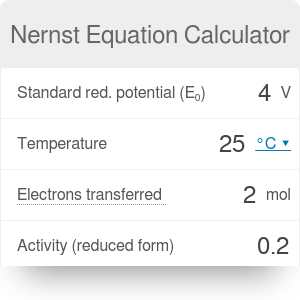Standard red. potential (E₀)
V
Temperature
°F
Electrons transferred
mol
Activity (reduced form)
Activity (oxidized form)
Reduction potential (E)
V

# Nernst Equation Calculator

By Bogna Haponiuk

This Nernst equation calculator presents the fundamental formula of electrochemistry - the Nernst equation, also known as the cell potential equation. It is a formula that allows you to calculate the reduction potential of a half-cell or full cell reaction. If you don't know what is the reduction potential, don't worry - we will explain all terms, describe in detail how to calculate the cell potential, and finish with a Nernst equation example that best presents its application.

## What is the reduction potential?

Reduction potential of a half-cell or full cell reaction is also called the redox potential or oxidation/reduction potential. It measures the tendency of molecules (or atoms, ions etc.) to acquire electrons and hence be reduced. This value is measured in volts (V) - the same units that are used by our Ohm's law calculator.

Why exactly is it called oxidation/reduction potential? Oxidation occurs when electrons are removed - for example when a free radical steals an electron from a cell. Reduction, on the other hand, means receiving or gaining electrons, for instance when an antioxidant donates an electron to a free radical.

What does it mean in terms of reduction potential? A solution with a higher potential will have a tendency to gain electrons (be reduced), and a solution with a lower potential - to lose electrons (be oxidized). Note that a high reduction potential doesn't mean that the reaction will occur - the reaction still requires some activation energy to be supplied.

It is difficult to measure the absolute potential of a solution. That's why the reduction potentials are usually defined relative to a reference electrode.

The standard reduction potential is the redox potential measured under standard conditions: 25°C, activity equal to 1 per ion, and pressure of 1 bar per gas participating in the reaction. The standard reduction potential is defined relative to a standard hydrogen electrode(SHE), which is arbitrarily given a potential of 0 volts.

## What is the cell potential equation?

The Nernst equation (cell potential equation) relates the reduction potential to the standard electrode potential, temperature, and activities of molecules. Activities can be substituted by concentrations for an approximate result. For a half-cell or full cell reaction,

`E = E₀ - RT/zF * ln([red]/[ox])`

where:

• E is the reduction potential, expressed in volts (V);
• E₀ is the standard reduction potential, also expressed in volts (V);
• R is the gas constant, equal to 8.314 J/(K * mol);
• T is the temperature at which the reaction would occur, measured in Kelvins (K);
• z is the number of moles of electrons transferred in the reaction (mol);
• F is the Faraday constant, equal to the number of coulombs per mole of electrons (96 485.3 C/mol);
• [red] is the chemical activity of the molecule (atom, ion...) in the reduced form. It can be substituted by concentration;
• [ox] is the chemical activity of the molecule (atom, ion...) in the oxidized form. It also can be substituted by concentration.

## How to calculate reduction potential: a Nernst equation example

We will use the Nernst equation calculator to find the reduction potential of a cell basing on the following reactions:

`Mg → Mg2+ + 2e-`, where E₀ = +2.38 V

`Pb2+ + 2e- → Pb`, where E₀ = -0.13 V

1. First, we need to write down the total reaction and calculate the total standard redox potential:

`Pb2+(aq) + Mg(s) → Mg2+(aq) + Pb(s)`

`E₀(cell) = 2.38 V + -0.13 V = 2.25 V`

1. The next step is to determine the temperature and number of moles of electrons. We can assume the temperature to be equal to 25°C. 2 moles of electrons were transferred in this reaction.
2. Now, we need to decide which molecules are oxidized and which reduced. The ones that gain electrons - that is, lead (Pb) molecules - are reduced. The magnesium (Mg) molecules are oxidized.
3. The last step before before performing calculations is to determine the proportion of activity or concentration. If we know the concentration of Pb molecules to be 0.200 M and of magnesium to be 0.020 M, the the concentration proportion is `[red]/[ox] = [Mg2+]/[Pb2+] = 0.020/0.200 = 0.1`.
4. Now we can combine all of these results and introduce them into the Nernst equation calculator. The final value of reduction potential is equal to 2.28 V.
Bogna Haponiuk

## Get the widget!

Nernst Equation Calculator can be embedded on your website to enrich the content you wrote and make it easier for your visitors to understand your message.

It is free, awesome and will keep people coming back!